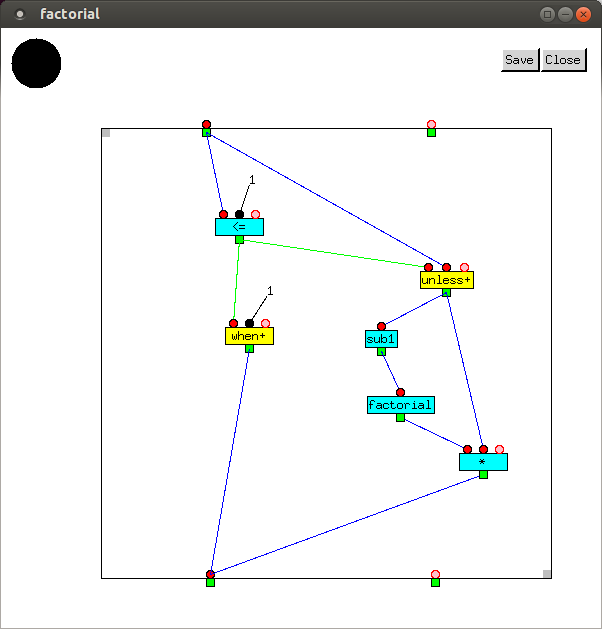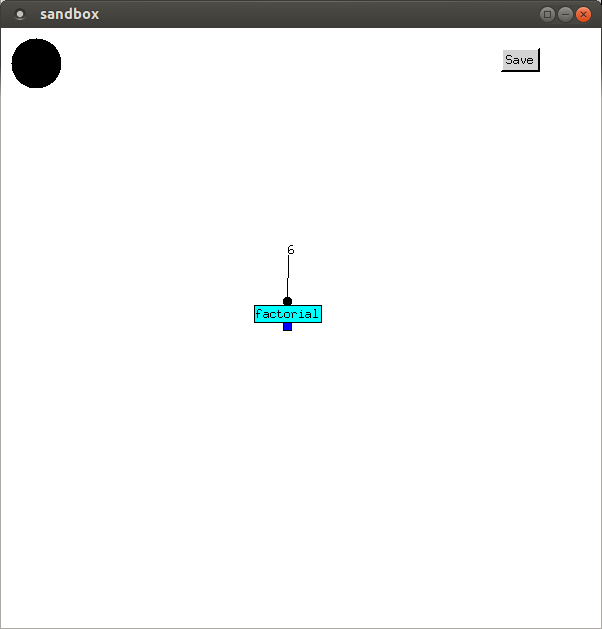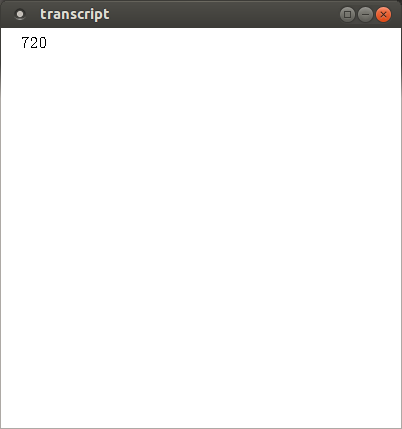# Recursive Functions

 Most programmers learn iteration first, and many find it hard to get their heads around recursion. However, because recursion in Full Metal Jacket is similar to recursion in other programming languages, and iteration is quite different, recursion is explained here first. Recursive functions contain, and rely upon, a conditional expression with several clauses. If certain conditions are satisfied, the function calls itself, but with simpler (i.e. reduced) arguments; otherwise, a value is returned directly. `factorial` is a simple example of a recursive function. If its input is less than or equal to 1, it returns 1; otherwise it returns the product of its input and the factorial of its input minus 1: ```factorial(n) = if n <= 1 then 1 else n * factorial(n-1) ``` There are several other ways of defining factorial, for example using tail recursion or iteration. In Full Metal Jacket, `factorial` is:If the input is 1 or below, 1 is output by the `when+` vertex. Otherwise, 1 is subtracted from the input value by the `sub1` vertex, and this is the input for a recursive call to `factorial`, the result of which is multiplied by the input value and sent to the outgate, which returns it. Incidentally, Common Lisp calls the function to subtract 1 `1-`. More sensibly, Scheme calls it `-1+`. It is not the same as the `--` in C, C++, and Java, which destructively modifies its input.© Copyright Donald Fisk 2015, 2016